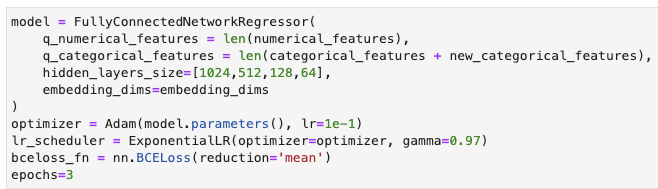Hi

I’m training a Fully Connected NN with Pytorch, and the model seems to perform very well. this is the model, and the hyper-parameters:

The Model (I’ve not changed the name to `FullyConnectedNetworkClassifier`)

As you can see, the output layer has a `sigmoid`, so my model predicts a probability

The hyper-parametersThe results

I made a terrible mistake. I didn’t realize that the both dataset’s and unbalanced. The column target has two values, `True` or `False`, and, as I said, the unbalancing comes in the form that in both dataset’s the samples with `target=False` are the 90% (10% of samples with `target=True`) of the total (both in training and validation)

So my questions:

1. My model, as it’s, … is it predicting the probability of the `True` class (`target=True`), or the False one (`target=False`)?
2. How can I parametrize `BCELoss` with `weights`? how much should be the magnitud of those `weights`? What is the in intuition of the `weight` parameter, exactly?
3. Could I use `BCEWithLogitsLoss` to work with my unbalanced dataset’s? what about its `weight` and `pos_weight` parameters?

Best regards and thanks

1. Most likely your model is predicting the majority class only, so I guess it’s predicting the `False` class. You can check it by printing the unique predictions for the datasets.

2. You could use `nn.BCEWithLogitsLoss`, remove the `sigmoid`, and set the `pos_weight` as `number_negative_samples / number_positive_samples`. If that doesn’t work, try to use `WeightedRandomSampler` as described here.

3. see 2.

Also, note that the accuracy can be misleading for an imbalanced dataset as described in the Accuracy paradox.

Most likely your model is predicting the majority class only, so I guess it’s predicting the `False` class. You can check it by printing the unique predictions for the datasets.

You mean, take the predictions and see their probability distributions?

You could use `nn.BCEWithLogitsLoss` , remove the `sigmoid` , and set the `pos_weight` as `number_negative_samples / number_positive_samples` . If that doesn’t work, try to use `WeightedRandomSampler` as described here.

I’ll do it and let you know

Also, note that the accuracy can be misleading for an imbalanced dataset as described in the Accuracy paradox.

Thanks @ptrblck, best regards

Yes, exactly. You could use a threshold of e.g. `0` to create the predictions and check the predicted class labels (similar to how you would calculate the accuracy). I assume `torch.unique` would return only the majority class.

Ok, right … one more thing to test this weekend:

1. Do you think @ptrblck that the use of embeddings and the fact that the model get trained in batches lessens the unbalanced situation? My model, as it’s, in the unseen test_set had a roc_auc of 86,7%, which puts me in third position in an internal competition in my job.

Best regards

No, I don’t think using batches would reduce the imbalance.
E.g. if you are passing the complete dataset in a single batch, the imbalance would still be there.
Of course you could use the `WeightedRandomSampler` to create balanced batches.

Ok …

In a couple of hours more start my happy coding hours, which extends during the weekend. I’ll be testing all the things you’ve suggested me … so, stay tune plsHere in Chile is 20.16 PM … by the way … Where are you writting from?

Best regards @ptrblck